Courses

# RCC Design - 2

## 10 Questions MCQ Test GATE Civil Engineering (CE) 2022 Mock Test Series | RCC Design - 2

Description
This mock test of RCC Design - 2 for Civil Engineering (CE) helps you for every Civil Engineering (CE) entrance exam. This contains 10 Multiple Choice Questions for Civil Engineering (CE) RCC Design - 2 (mcq) to study with solutions a complete question bank. The solved questions answers in this RCC Design - 2 quiz give you a good mix of easy questions and tough questions. Civil Engineering (CE) students definitely take this RCC Design - 2 exercise for a better result in the exam. You can find other RCC Design - 2 extra questions, long questions & short questions for Civil Engineering (CE) on EduRev as well by searching above.
QUESTION: 1

### As per IS: 456 in a two – way slab, the width of the middle strip along each span should be:

Solution:

See figure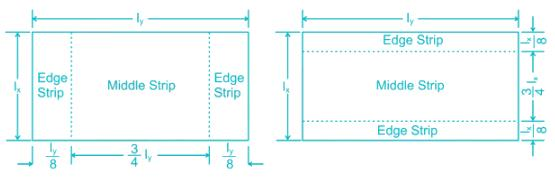QUESTION: 2

### The appropriate expression in assessing development length is:

Solution:

A development length is the amount of rebar length that is needed to be embedded or projected into concrete to create desired bond strength between the two materials and also to develop required stress in steel at that section. It is given by: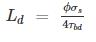QUESTION: 3

### For bars in tension, a standard hook has an anchorage value equivalent to a straight length of

Solution:

As per IS 456: 2000,

A standard hook has an anchorage value equivalent to a straight length of 16ϕ.

The anchorage value of standard U-type hook shall be 16 times the diameter of the bar.

The anchorage value of standard bend shall be considered as 4 times the diameter of the bar for each 45o bend subject to a maximum value of 16 times the diameter of the bar.

QUESTION: 4

A floor slab of thickness t is cast monolithically transverse to a rectangular continuous beam of span L and width B. If the distance between two consecutive points of contra flexure is, L0, the effective width of compression flange at continuous support is

Solution:

For T-beams effective width of compression flange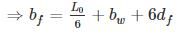For L-beam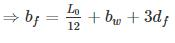QUESTION: 5

Clear distance between lateral restrains for continuous reinforced concrete beam of size 250 × 500 mm (effective depth) according to IS 456:2000 shall be limited to:

Solution:

For simply supported and continuous beam:

clear distance between lateral restrains shall not be greater than: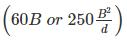which ever is lesser

Where,

B = width of the beam = 250 mm

d = effective depth of the beam = 500 mm

i) 60 B = 60 × 250 = 15000 mm = 15 m
ii)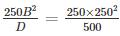= 31250 mm = 31.25m
lesser will be 15 m.

QUESTION: 6

It is proposed to reduce 20 mm diameter bar to 16 mm diameter in an axially loaded column. Grade of steel used is Fe500. Which one of the following lap length should be used if the design bond stress for M25 grade concrete is 1.4 MPa.

Solution:

In compression zone,

Lap Length = Maximum of development length or 24 ϕ (where ϕ is the dia of smaller dia bar)

Development length,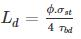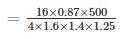[60% increase in bond stress as HYSD bar]

25% increase in bond stress due to compression.

= 621.43 mm

Now 24ϕ = 24 × 16 = 384 mm

So the lap length should be 621.43 mm

QUESTION: 7

For a two way simply supported RC slab of size 3 m × 9 m effective span. The maximum spacing of distribution bars satisfying codal provisions as per IS 456 : 2000 is?

M-20 concrete and Fe-415 steel grade is used in the slab

Solution:

Maximum spacing of distribution bars in slab is = 5d or 450 mm (whichever is lesser)

d → effective depth of slab

A – value for simply supported slab for HYSD bars = 0.8 × 35 = 28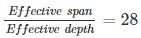Use shorter span for calculation

3000/28 = deff

deff  = 107.14 mm

Maximum spacing:

I. 5 × 107.14 = 535.7

II. 450 mm

Use lesser of above two

so, maximum spacing = 450 mm

QUESTION: 8

A cantilever steel beam of 3 m span carries a uniformly distributed load of 20 kN/m inclusive of self-weight. The beam comprises ISLB200@198 N/m, flange 100 mm × 7.3 mm, web thickness 5.4 mm, Ixx = 1696.6 cm4, lyy = 115.4 cm4. Bending and shear stresses in the beam are respectively.

Solution: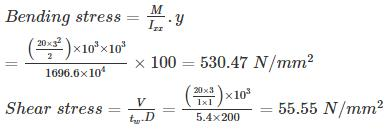QUESTION: 9

At limit state of collapse, an RC beam is subjected to flexural moment 200 kN.m, shear force 20 kN and torque 15 kN.m. The beam is 400 mm wide and 500 mm effective depth with 50 mm cover. The equivalent shear force and equivalent moment is :

Solution: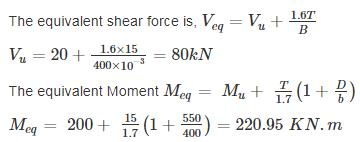QUESTION: 10

A rectangular beam of 500mm × 800mm with an effective cover of 40 mm is subjected to a factored shear force 30 KN, a bending moment of 100 KN - m and a torsional moment of 15 KN - m. The design bending moment in KN - m for the beam will be ________

(Use shear resistance of the cross-section τc is 0.48 MPa)

Solution: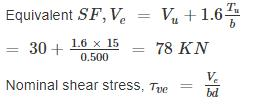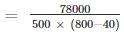= 0.205 N/mm2 < τc

So the design bending moment will be, Mu = 100 KN - m.

• RCC - 2